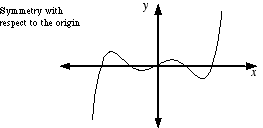index: click on a letter A B C D E F G H I J K L M N O P Q R S T U V W X Y Z A to Z index index: subject areas numbers & symbols sets, logic, proofs geometry algebra trigonometry advanced algebra & pre-calculus calculus advanced topics probability & statistics real world applications multimedia entrieswww.mathwords.com about mathwords website feedback

 Symmetric about the Origin Symmetric across the Origin Symmetric with Respect to the Origin Describes a graph that looks the same upside down or right side up. Formally, a graph is symmetric with respect to the origin if it is unchanged when reflected across both the x-axis and y-axis.See also